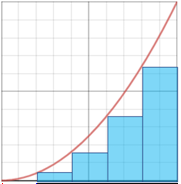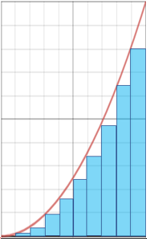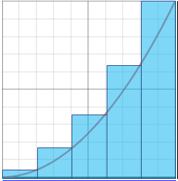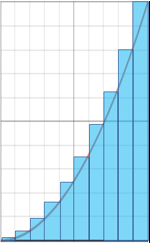# Riemann sum

A Riemann sum is a method used for approximating an integral using a finite sum. In calculus, the Riemann sum is commonly taught as an introduction to integrals, and is used to estimate the area under a curve by partitioning the region into shapes similar to the region being measured, the area of which can be calculated. The area of these shapes are then added to esstimate the area under the curve. Since the shapes being used won't exactly match the shape of the region there will be some error. This error can be reduced by using a smaller width, dividing the region more finely. The smaller the width of the shape, the more accurate the estimation. Two of the most basic methods of calculating Riemann sums are the left Riemann sum and the right Riemann sum.

### Left Riemann sum

The left Riemann sum involves approximating a function through use of its left endpoint. Below is an example of a Riemann sum for f(x) = x2 between the points 0 and 1, with n = 5 and n = 10 using a left Riemann sum.n = 5n = 10

A left Riemann sum will result in an overestimation if f(x) is only decreasing on the given interval, such as it would be left of the y-axis of f(x) = x2. If f(x) is only increasing on the interval, such as it is in our example, the left Riemann sum results in an underestimation.

### Right Riemann sum

The right Riemann sum is similar to the left Riemann sum with the key difference being that the function is approximated using the right endpoint. Using f(x) = x2 between the points 0 and 1, with n = 5 and n = 10, the Riemann sum can be seen as follows:n = 5n = 10

The right Riemann sum results in an underestimation if f(x) is only decreasing on the given interval, and an overestimation, as in our example, when f(x) is only increasing on the interval.